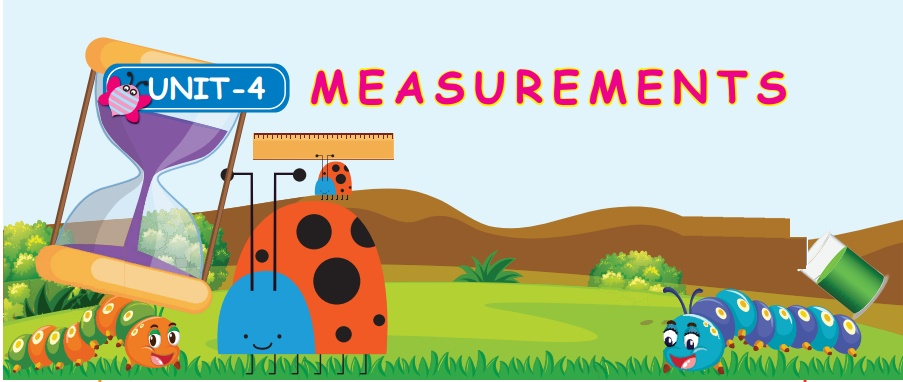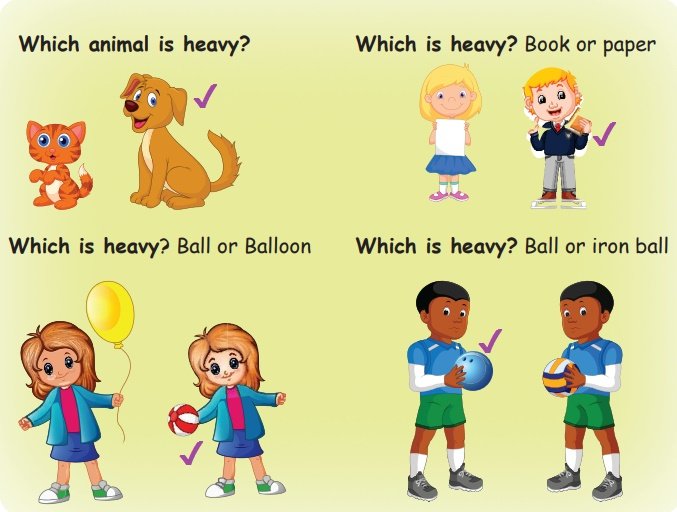Home | | Maths 4th Std | Introduction

# Introduction

Vegetables, fruits and rice are measured in kilogram (kg) ; Gold, pepper, Tea powder, silver, Coffee powder etc . are measured by grams.

UNIT − 4

MEASUREMENTSIntroduction

Nisha and Joe were friends. Nisha had a pet animal. It was a dog. It’s name was Arjun. It was 25 kg weight. Joe had a pet animal. It was a cat. It’s name was pooja. It was 15 kg weight.Which animal is heavy? Dog

Which is heavy? Book or paper

Which is heavy? Ball or Balloon

Which is heavy? Ball or iron ball

Activity

Estimate and tick the correct option

Find the weight whether it is in gram or kilogram.Different weighing instruments are used to measure weight.Common balance, Digital balance, Weigh machine, Spring balance

Note: Vegetables, fruits and rice are measured in kilogram (kg)

Activity

Observe the given picture and circle the correct weight.Let us know the Weighing stones are in grams and kilogramsgram can be written as “g”

Note: Gold, pepper, Tea powder, silver, Coffee powder etc . are measured by grams.1 kilogram = 1000 grams

Change into grams and kilogram

a) 1 kg = 1000 g

b) 2 kg = 2000 g

c) 3 kg = 3000 g

d) 15 kg = 15000 g

e) 23 kg = 23000 g

Tags : Measurements | Term 2 Chapter 4 | 4th Maths , 4th Maths : Term 2 Unit 4 : Measurements
Study Material, Lecturing Notes, Assignment, Reference, Wiki description explanation, brief detail
4th Maths : Term 2 Unit 4 : Measurements : Introduction | Measurements | Term 2 Chapter 4 | 4th Maths